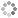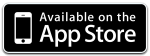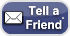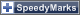Loading...

Enter your formula and the range:

Available functions: sin, cos, tan, asin, acos, atan, sqrt, exp, log, pow, abs, round, ceil, floor, random, PI, E
 Examples y(x) = x-min = x-max =
Hint: Draw more than one function by separating formulas by semicolons